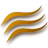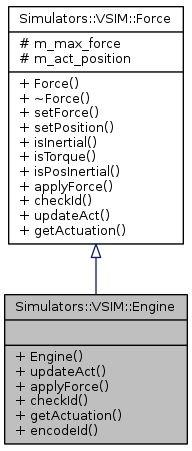DUNE: Uniform Navigational Environment  2016.05.0
Simulators::VSIM::Engine Class Reference

## Detailed Description

Engine class responsible to model propellers.

## Public Member Functions

Engine (unsigned int id=0, double aforce=0, double pos=0, double orientation=0, float force=0)

void updateAct (double value)

void applyForce (double speed, double forces)

bool checkId (unsigned int testid)

double getActuation (void)Public Member Functions inherited from Simulators::VSIM::Force
Force (void)

virtual ~Force (void)

void setForce (double, double, double, bool, bool)

void setPosition (double, double, double, bool)

bool isInertial (void)

bool isTorque (void)

bool isPosInertial (void)

## Static Public Member Functions

static int encodeId (int id)Protected Attributes inherited from Simulators::VSIM::Force
double m_max_force 

double m_act_position 

## Constructor & Destructor Documentation

 Simulators::VSIM::Engine::Engine ( unsigned int id = `0`, double aforce = `0`, double pos = `0`, double orientation = `0`, float force = `0` )

Engine constructor.

Parameters
 [in] id engine id. [in] aforce force distribution across three axes. [in] pos position of the engine relative to the center of the vehicle. [in] orientation orientation of the engine. [in] force maximum force of the engine.

## Member Function Documentation

 void Simulators::VSIM::Engine::applyForce ( double speed, double forces )
virtual

Apply engine's force.

Parameters
 [in] speed speed reference of the vehicle. [out] forces control forces to be added to the vehicle.

Reimplemented from Simulators::VSIM::Force.

 bool Simulators::VSIM::Engine::checkId ( unsigned int testid )
virtual

Check engine's id.

Parameters
 [in] testid engine id.
Returns
true if id matches, false otherwise.

Reimplemented from Simulators::VSIM::Force.

 static int Simulators::VSIM::Engine::encodeId ( int id )
inlinestatic

Encode id function.

Referenced by Simulators::VSIM::Vehicle::updateEngine().

 double Simulators::VSIM::Engine::getActuation ( void )
virtual

Return vehicle actuation.

Returns
vehicle actuation.

Reimplemented from Simulators::VSIM::Force.

 void Simulators::VSIM::Engine::updateAct ( double value )
virtual

Update engine's actuation.

Parameters
 [in] value actuation value.

Reimplemented from Simulators::VSIM::Force.

Collaboration diagram for Simulators::VSIM::Engine: